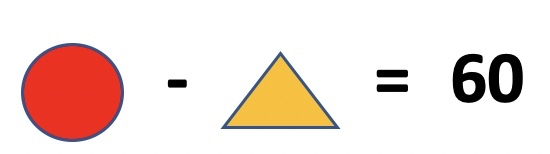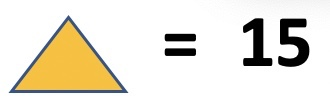# Olympiad Math Preparation – Class 2

## Addition of 2 and 3 digit numbers ( without regrouping/carry )

1. The sum of greatest and smallest number is _________________ . Numbers : 188, 508, 345, 801, 290

2. Select the correct option

3. The sum of numbers shown in two abacuses below is ________________

4. Ifandthen what is value of?

5. Ifis added to 100, the result is 150. Thenis _________.

6. What is sum of largest and smallest 3 digit number ?

7. Duggu has 54 chocolates. Dakshu has 45 chocolates. How many chocolates both of them have ?

8.and. Find the value of